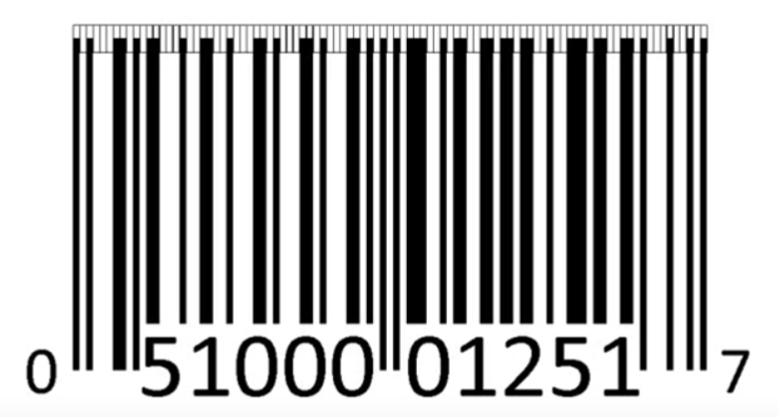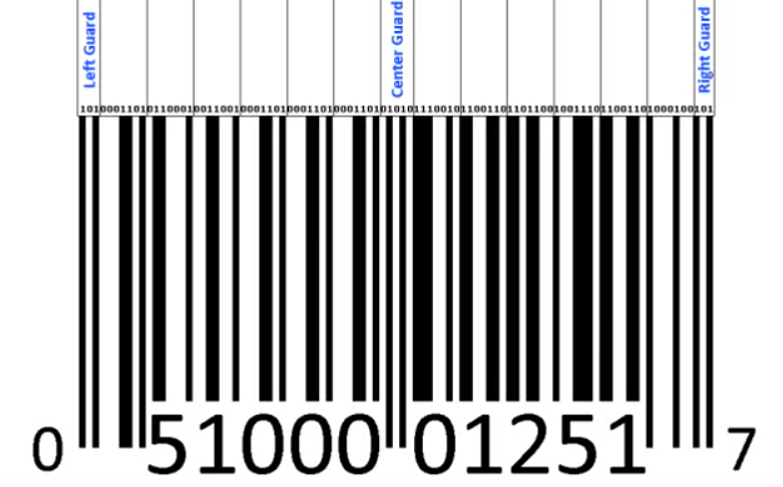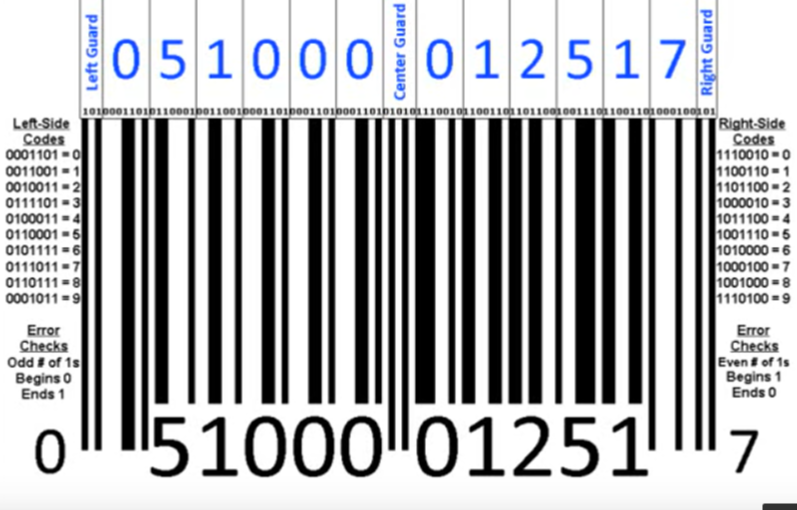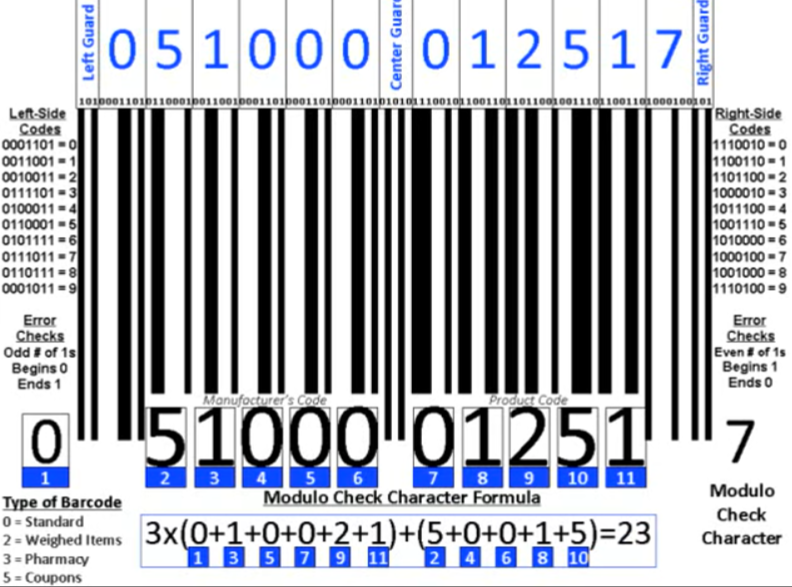Select Page

9

FEBRUARY, 2017

Barcodes are found in virtually all products that you see in any store. When a laser from a computer scans a barcode, it is actually scanning through a series of 95 evenly spaced columns and checking to see if each one of those columns is reflecting a lot of laser light or virtually none.## How Do Computers read/understand Barcodes?

Computers only understand ones and zeros so any of the columns that reflect virtually no light are considered a one, and any of the columns that reflect a lot of light are considered 0, probably the reverse of what you might expect. The computer begins reading the columns from left to right. The computer continues reading the columns all the way across the bar code and comes up with a number that is 95 digits long full of ones and zeros. These ones and zeros are then grouped into 15 different sections. 12 of these sections are used for the numbers at the bottom of the barcode. The other three are used as guards.These guards let the computer know where the bar code begins and ends and also where the 6 numbers on each side begin and end. This is important because the numbers on the left are identified based upon one set of codes and the numbers on the right are identified based upon another set of codes. These codes are different because the computer needs to know whether it is reading the bar code from left to right or whether the bar code is being read upside down. It figures that out based upon how many ones there are in the code for each digit.

The codes on the Left always have an odd number of ones and the codes on the right always have an even number of ones. If the computer reads an even number of ones on the left hand side it knows that the bar code is flipped upside down and once it reads it, it can just flip the numbers around before processing them and also has an additional air check.All the codes on the left side begin with a zero and end with one and all the codes on the right side begin with the one and end with a zero. In this figure above case you will see that those numbers are the exact same as the numbers below. what do these numbers actually mean?

The first number on the left hand side (the 0) which is outside of the actual barcode tells us what type of bar code this is. A 0 is a standard barcode, a 2 is a weight item like fruit or meat and 3 is a pharmacy item and the 5 is a coupon.

The next set of five numbers tells us who the manufacturer of the product is. The second set of five digits tells us the product code. Finally the last number on the right hand side is called the modulo check character. This is another form of error checking.

## How does the computer know that it processed everything correctly?

When the computer scans the barcode and processes the numbers it needs a final way to know that it read and processed everything correctly so it performs a calculation and comes up with the modulo check character. The modulo check character formula is actually based upon the positions of each of the numbers at the bottom of the barcode.

It first adds up the digits and the odd number of positions, and then it adds up the digits in the even number positions. It then multiplies the odd number of digits by three and adds that amount to the sum of the even number of digits.We will go ahead and plug in the numbers from our barcode and we come up with a final total of 23. We then subtract the result of this formula from the next highest multiple of 10 to get the modulo check character. So the next highest multiple of 10 after 23 is 30, and 30 – 23 is seven. This way we know that we have read the barcode correctly.